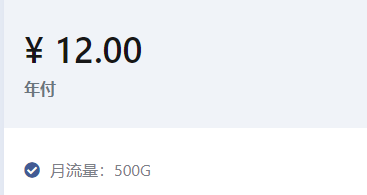• 累计撰写 173 篇文章
• 累计创建 3 个标签
• 累计收到 115 条评论

### 目 录CONTENT# 18-类与接口.md2022-03-21 / 0 评论 / 0 点赞 / 593 阅读 / 2,943 字 / 正在检测是否收录...广告

# 18-类与接口

## 类实现接口§

interface Alarm {
}

class Door {
}

class SecurityDoor extends Door implements Alarm {
}
}

class Car implements Alarm {
}
}


interface Alarm {
}

interface Light {
lightOn(): void;
lightOff(): void;
}

class Car implements Alarm, Light {
}
lightOn() {
console.log('Car light on');
}
lightOff() {
console.log('Car light off');
}
}


## 接口继承接口§

interface Alarm {
}

interface LightableAlarm extends Alarm {
lightOn(): void;
lightOff(): void;
}


## 接口继承类§

class Point {
x: number;
y: number;
constructor(x: number, y: number) {
this.x = x;
this.y = y;
}
}

interface Point3d extends Point {
z: number;
}

let point3d: Point3d = {x: 1, y: 2, z: 3};


class Point {
x: number;
y: number;
constructor(x: number, y: number) {
this.x = x;
this.y = y;
}
}

const p = new Point(1, 2);


class Point {
x: number;
y: number;
constructor(x: number, y: number) {
this.x = x;
this.y = y;
}
}

function printPoint(p: Point) {
console.log(p.x, p.y);
}

printPoint(new Point(1, 2));


class Point {
x: number;
y: number;
constructor(x: number, y: number) {
this.x = x;
this.y = y;
}
}

interface PointInstanceType {
x: number;
y: number;
}

function printPoint(p: PointInstanceType) {
console.log(p.x, p.y);
}

printPoint(new Point(1, 2));


class Point {
x: number;
y: number;
constructor(x: number, y: number) {
this.x = x;
this.y = y;
}
}

interface PointInstanceType {
x: number;
y: number;
}

// 等价于 interface Point3d extends PointInstanceType
interface Point3d extends Point {
z: number;
}

let point3d: Point3d = {x: 1, y: 2, z: 3};


class Point {
/** 静态属性，坐标系原点 */
static origin = new Point(0, 0);
/** 静态方法，计算与原点距离 */
static distanceToOrigin(p: Point) {
return Math.sqrt(p.x * p.x + p.y * p.y);
}
/** 实例属性，x 轴的值 */
x: number;
/** 实例属性，y 轴的值 */
y: number;
/** 构造函数 */
constructor(x: number, y: number) {
this.x = x;
this.y = y;
}
/** 实例方法，打印此点 */
printPoint() {
console.log(this.x, this.y);
}
}

interface PointInstanceType {
x: number;
y: number;
printPoint(): void;
}

let p1: Point;
let p2: PointInstanceType;


0
1.2.广告
• 0
1.2.